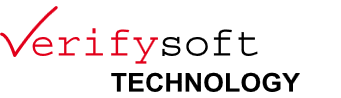# Example of Condition coverage

## Simple example

Assume we want to test the following code extract:

```        ```
if ( (A || B) && C )
{
/* instructions */
}
else
{
/* instructions */
}
```

```

where A, B and C represent atomic boolean expressions (i.e. not divisible in other boolean sub-expressions).

In order to ensure Condition coverage criteria for this example, A, B and C should be evaluated at least one time "true" and one time "false" during testes.

So, in our example, the 3 following testes would be sufficient to valid Condition coverage:

```      A = true  / B = not eval / C = false
A = false / B = true     / C = true
A = false / B = false    / C = not eval

```

## More complex example

Assume we replace the condition: `( (A || B) && C )`
by: `( ((u == 0) || (x>5)) && ((y<6) or (z == 0)) )`

A full Test Coverage would consist into building the following truth table and testing each combination:

 Test case n° A: (u == 0) B: (x>5) C: (y<6) D: (z == 0) ( (A || B) && (C || D) ) 1 F F F F F 2 F F F T F 3 F F T _ F 4 F T F F F 5 F T F T T 6 F T T _ T 7 T _ F F F 8 T _ F T T 9 T _ T _ T

On the other hand, to ensure Condition coverage, we should test (for example) just the 3 combinations here-before underlined in yellow.

Glossary:

Condition: a logical indivisible (atomic) expression. It is often called boolean variable, represented by a capital letter (A, B, C, etc.), can only be equal to "true" or "false", but can not be divided in other simpler "sub-conditions".

Decision : a logical expression which can be composed of several conditions separated by logical operators like "or", "and", "xor".

The Test Coverage Analyzer Testwell CTC++ can do measurements for all kinds of test coverage criteria (particularly for MC/DC and MCC) requested for "critical software development" in order to get certifications, such as DO-178B of the Federal Aviation Administration (FAA).